Courses
Courses for Kids
Free study material
Offline Centres
More

# Geometrical Meaning of Zeroes of the PolynomialLast updated date: 29th Nov 2023
Total views: 80.1k
Views today: 0.80k## Geometrical Meaning of Zeroes of the Polynomial : A Detailed Summary

Expression consists of variables, coefficients, and arithmetic operations. An expression may consist of more than one variable, and the variable's power is any real number.

Let’s understand polynomials definition with example; a polynomial is an expression of one variable. The power of variables of a polynomial must be a natural number. A polynomial represented by P(x), where x is the variable of the polynomial. For example P(x) = $2x^3+5x+3$.

## Degree of a Polynomial

The degree of a polynomial is the highest power of the variable of the polynomial. The degree of linear polynomials is 1 because the highest degree of the polynomial is 1. The degree of a quadratic polynomial is 2 because the highest degree of the polynomial is 2. The degree of a cubic polynomial is 3 because the highest degree of the polynomial is 3.

## Types of Polynomials

The type of polynomial depends on the degree of the polynomial.

The general form of a polynomial is $P(x) = a_{n}x^{n}+a_{n-1}x^{n-1}+\cdots +a_1x+a_{0}$

• Constant polynomial: If $a_{n}=a_{n-1}=\cdots =a_2=a_{1}=0$ and $a_{0}\ne 0$, then the polynomial is known as a constant polynomial. For example: P(x) =3.

• Zero polynomial: If $a_{n}=a_{n-1}=\cdots =a_2=a_{1}=a_{0}= 0$, then the polynomial is known as a zero polynomial.

• Linear polynomial: If $a_{n}=a_{n-1} = a_2 = 0$ and $a_{1}\ne 0$, then the polynomial is known as linear polynomial.

• Quadratic polynomial: If $a_{n}=a_{n-1} =\cdots =a_3=0$ and $a_{2}\ne 0$, then the polynomial is known as quadratic polynomial.

• Cubic polynomial: If $a_{n}=a_{n-1} =\cdots0= a_4$ and $a_{3}\ne 0$, then the polynomial is known as quadratic polynomial.

## Zeroes of the Polynomial Definition

The zero of a polynomial is the value of the variable for which the value of the polynomial becomes zero.

The polynomial P(x) has a zero at x = a. This means the value of P(x) at x = a is zero that is P(a) = 0.

Assume a polynomial, $P(x)=2x+4$

Now equate P(x) with zero.

P(x) = 0

$\Rightarrow 2x+4=0$

$\Rightarrow 2x=-4$

$\Rightarrow x=-2$

The zero of the polynomial P(x)=2x+4 is x = -2.

## Number of Zeros of a Polynomial

• The degree of a linear polynomial is 1. Thus the number of zeros of a linear polynomial is at most 1.

• The degree of a quadratic polynomial is 2. Thus the number of zeros of a linear polynomial is at most 2.

• The degree of a cubic polynomial is 3. Thus the number of zeros of a linear polynomial is at most 3.

## How to Obtain the Zero of a Linear Polynomial Geometrically?

The degree of a polynomial is the highest power of the variable of the polynomial. The degree of a linear polynomial is 1. Thus the number of zeros of the polynomial is 1.

To get a zero of linear polynomials geometrically, we will find the point where the given linear polynomial cuts the x-axis. Suppose the polynomial cuts the x-axis at x = a, then the zero of the polynomial is a.

For Example,

Assume an equation of a linear polynomial P(x) = x+4.

The graphical representation of a linear polynomial is shown below:Linear polynomial

The linear polynomial P(x) cuts the x-axis at (-4,0). Thus the zero of the polynomial is -4. It means P(-4) = 0. The zero of the polynomial P(x) = x+4 is -4.

Since the given polynomial is a linear polynomial, thus it intersects the x-axis at a point.

## Geometrical Meaning of the Zeros of a Polynomial

The number of zeros of a polynomial is dependent on the degree of that polynomial. The number of zeros of a polynomial is less than or equal to the degree of the polynomial. The number of zeros of a polynomial is equal x-intercepts of the polynomials.

## Geometrical Representation of Zeros

The number of zeros is equal to the number of the x-intercept of the polynomial graph. Below we have the geometrical meaning of zeroes of polynomials with examples

• Linear polynomial: The number of the x-intercepts is 1. Thus, the number of zeros is 1 for the linear polynomial.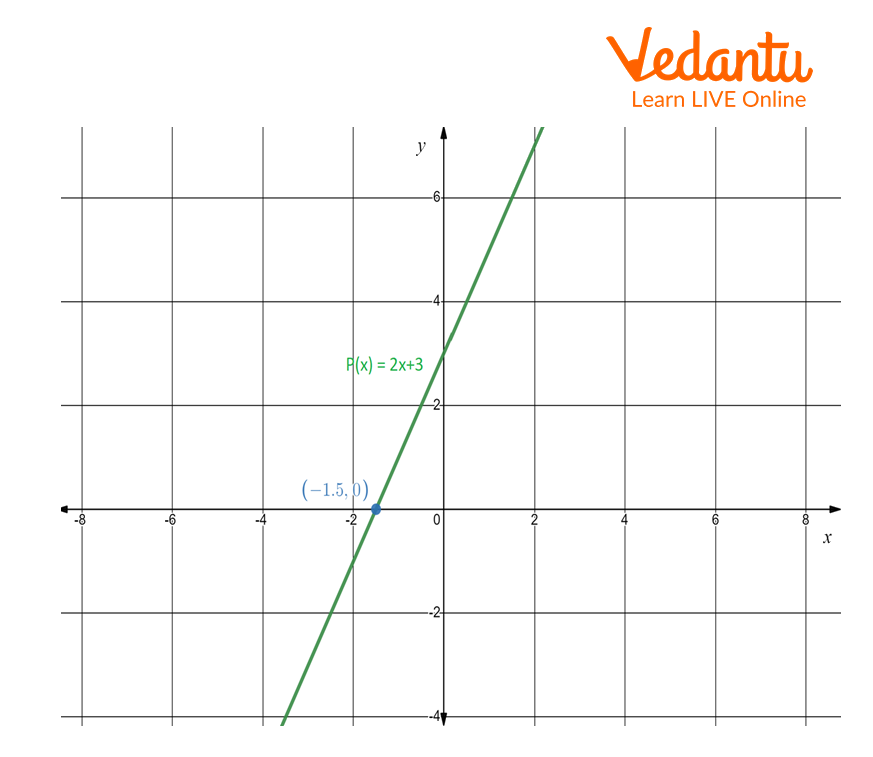Graph of a linear polynomial

• Quadratic polynomial: The quadratic polynomial has at most 2 zeros. Thus, the graph of quadratic polynomial intercepts at two points.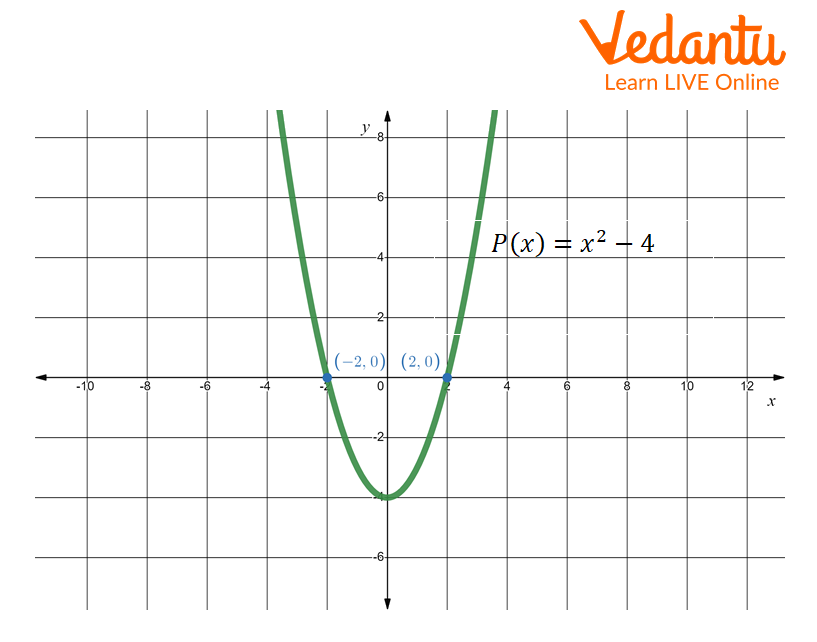• Cubic polynomial: The cubic polynomial has at most 3 zeros. Thus, the graph of cubic polynomial intercepts at three points.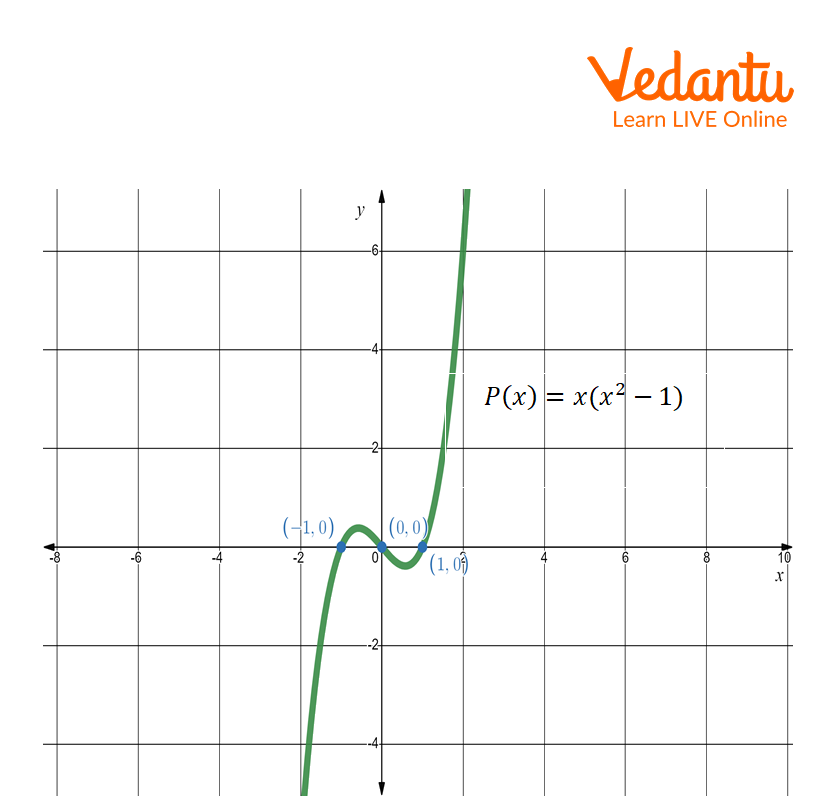Graph of a cubic polynomial

## Solved Problems

Q1 Find the number of zeroes of the polynomial in a given graph.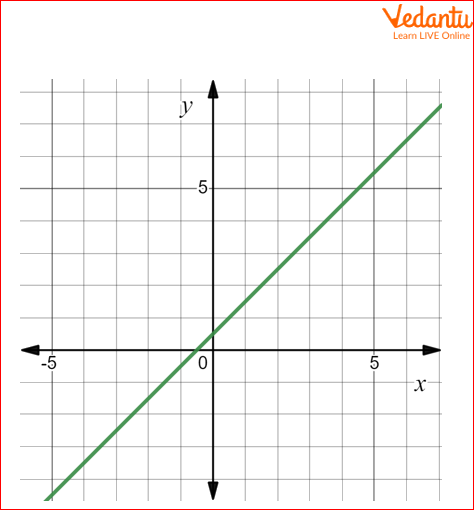Solution 1: From the above geometrical presentation we can see that the straight line intersects the x-axis at one time, therefore the number of zeros in the given polynomial is one.

Q2 Find the zeros of the polynomial $P(x) =4x^{2}-16$.

Solution 2: Given polynomial is $P(x) =4x^{2}-16$.

Equate P(x) with zero:

$4x^{2}-16=0$

$\Rightarrow 4x^{2}=16$

Divide both sides by 4:

$\Rightarrow x^{2}=4$

Take square root both sides

$\Rightarrow x=\pm 4$

The zeros of the polynomial are $\pm 4$.

Q3 Find out the zero of the polynomial y=x+1 geometrically.

Solution3: First we make the table for y=x+1

 x -2 -1 0 1 2 3 4 5 6 y=x+1 -1 0 1 2 3 4 5 6 7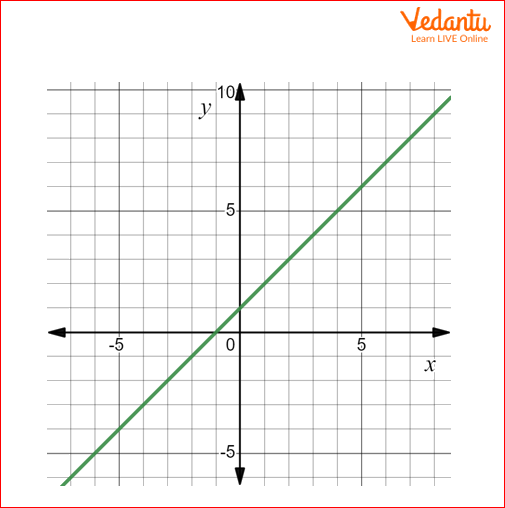Graph for given table

We can see that at x=-1, given straight line intersecting the x-axis, therefore x=-1 is zero of the given polynomial.

## Interesting Facts

• If the zeros of a quadratic polynomial are the same, then the graph of the quadratic polynomial touches the x-axis at a point.

• If the zeros of a cubic polynomial are the same, then the graph of the cubic polynomial cuts the x-axis at a point.

## Summary

The number of zeros of a polynomial depends on the degree of the polynomial. The number of zeros of a polynomial equals the number of x-intercepts of the polynomial. If P(a) = 0, then a is a zero of the polynomial P(x). A linear polynomial has at most 1 zero. A quadratic polynomial has at most 2 zeros and a cubic polynomial has at most 3 zeros.

## Practice Problem

Q1 Write the type of the given polynomial (1) 2x+3 (2) $2x^{2}+3$

Solution: (1) linear polynomial (2) Quadratic polynomial

Q2 From the given table find the zero of the polynomial.

 X 3 2 1 0 -1 Y=X-2 1 0 -1 -2 -3

Solution: The zero is X = 2

## FAQs on Geometrical Meaning of Zeroes of the Polynomial

1. What are the parts of a polynomial?

The different parts of a polynomial are:

• Terms: Each monomial is part of the polynomial.

• Coefficients: The numbers that accompany each term of the polynomial.

• Degree: The largest exponent to which the polynomial variable is raised.

• Variable: It is the letter that has the polynomial.

2. What is the difference between zero of the polynomial and zero polynomial?

A zero of a polynomial is the value where the polynomial becomes zero.

For example: The zero of the polynomial P(x) = 4x + 2 is $- \dfrac{1}{2}$ , because $P\left( { - \dfrac{1}{2}} \right) = 0$.

The zero polynomial is a special type of polynomial where the coefficient of ${x^n}$ is always zero, where n is a whole number.

For example P(x) = 0 is known as a zero polynomial.

3. Is it possible that 0 is a zero of a polynomial?

Assume a quadratic polynomial $P\left( x \right) = {x^2} - 4x$. Equate the polynomial with zero to find the zeros of the polynomial.

${x^2} - 4x = 0$

Solving the above equation

$x\left( {x - 4} \right) = 0$

Either,              or,

$x = 0$                   $x - 4 = 0$

The zeros of the polynomial are 0 and 4.

Thus, 0 can be a zero of a polynomial.